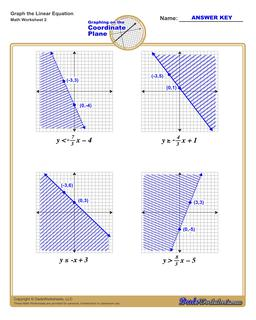PLEASE GO BACK AND USE THE BIG BLUE 'PRINT' BUTTON ON THE PAGE TO PRINT THE WORKSHEET CORRECTLY!Sorry for the trouble! The browser won't print the embedded worksheet PDF directly using the normal 'Print' command in the file menu, so you need to click the big 'Print' button to send just the worksheet and not the surrounding page to the printer.

# Math Worksheets: Linear Equations: Linear Equations: Graphing Linear Inequalities (Second Worksheet)## Graphing Linear Inequalities (Second Worksheet)

PropertyValue
DescriptionGraphing Linear Inequalities: Each graphing linear inequalities worksheet on this page has four coordinate planes and linear inequalities in slope-intercept form, and includes an answer key showing the correct graph. (Second Worksheet)
Resource TypeWorksheet